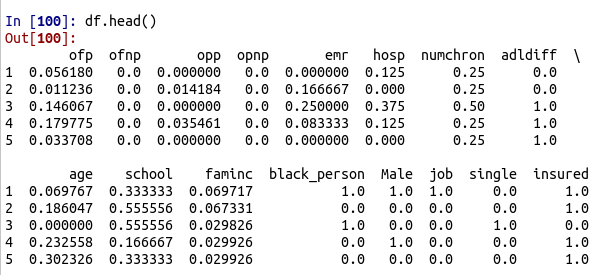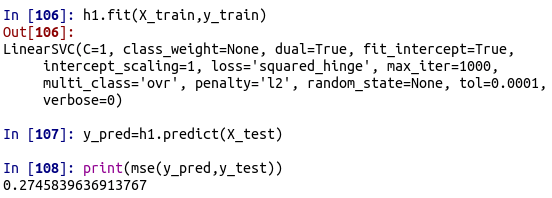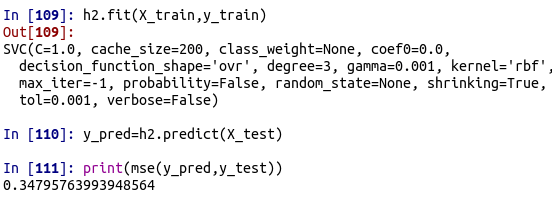# Support Vector Machines Regression with Python

This post will provide an example of how to do regression with support vector machines SVM. SVM is a complex algorithm that allows for the development of non-linear models. This is particularly useful for messy data that does not have clear boundaries.The steps that we will use are listed below

1. Data preparation
2. Model Development

We will use two different kernels in our analysis. The LinearSVR kernel and SVR kernel. The difference between these two kernels has to do with slight changes in the calculations of the boundaries between classes.

Data Preparation

We are going to use the OFP dataset available in the pydataset module. This dataset was used previously for classification with SVM on this site. Our plan this time is that we want to predict family inc (famlinc), which is a continuous variable.  Below is some initial code.

```import numpy as np
import pandas as pd
from pydataset import data
from sklearn import svm
from sklearn import model_selection
from statsmodels.tools.eval_measures import mse```

We now need to load our dataset and remove any missing values.

```df=pd.DataFrame(data('OFP'))
df=df.dropna()```

AS in the previous post, we need to change the text variables into dummy variables and we also need to scale the data. The code below creates the dummy variables, removes variables that are not needed, and also scales the data.

```dummy=pd.get_dummies(df['black'])
df=pd.concat([df,dummy],axis=1)
df=df.rename(index=str, columns={"yes": "black_person"})
df=df.drop('no', axis=1)

dummy=pd.get_dummies(df['sex'])
df=pd.concat([df,dummy],axis=1)
df=df.rename(index=str, columns={"male": "Male"})
df=df.drop('female', axis=1)

dummy=pd.get_dummies(df['employed'])
df=pd.concat([df,dummy],axis=1)
df=df.rename(index=str, columns={"yes": "job"})
df=df.drop('no', axis=1)

dummy=pd.get_dummies(df['maried'])
df=pd.concat([df,dummy],axis=1)
df=df.rename(index=str, columns={"no": "single"})
df=df.drop('yes', axis=1)

dummy=pd.get_dummies(df['privins'])
df=pd.concat([df,dummy],axis=1)
df=df.rename(index=str, columns={"yes": "insured"})
df=df.drop('no', axis=1)
df=df.drop(['black','sex','maried','employed','privins','medicaid','region','hlth'],axis=1)
df = (df - df.min()) / (df.max() - df.min())We now need to set up our datasets. The X dataset will contain the independent variables while the y dataset will contain the dependent variable

```X=df[['ofp','ofnp','opp','opnp','emr','hosp','numchron','adldiff','age','school','single','black_person','Male','job','insured']]
y=df['faminc']```

We can now move to model development

Model Development

We now need to create our train and test sets for or X and y datasets. We will do a 70/30 split of the data. Below is the code

`X_train,X_test,y_train,y_test=model_selection.train_test_split(X,y,test_size=.3,random_state=1)`

Next, we will create our two models with the code below.

```h1=svm.SVR()
h2=svm.LinearSVR()```

We will now run our first model and assess the results. Our metric is the mean squared error. Generally, the lower the number the better.  We will use the .fit() function to train the model and the .predict() function for test the modelThe mse was 0.27. This number means nothing only and is only beneficial for comparison reasons. Therefore, the second model will be judged as better or worst only if the mse is lower than 0.27. Below are the results of the second model.We can see that the mse for our second model is 0.34 which is greater than the mse for the first model. This indicates that the first model is superior based on the current results and parameter settings.

Conclusion

This post provided an example of how to use SVM for regression.